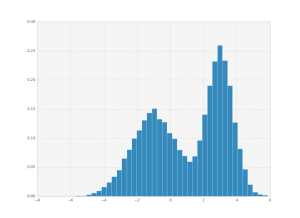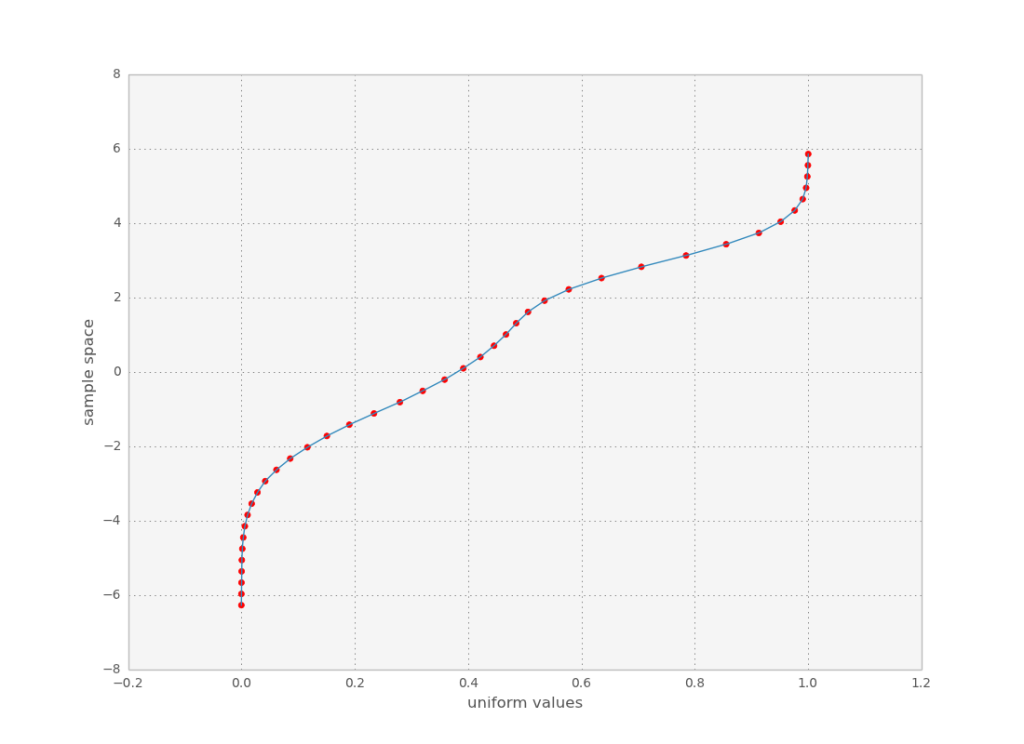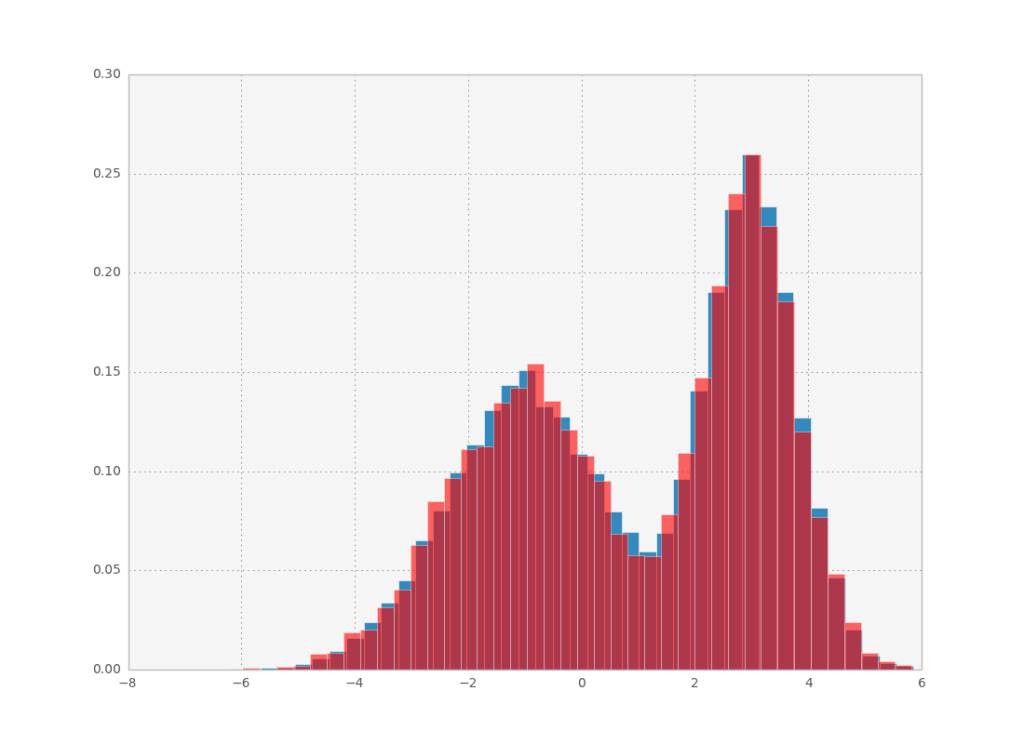# How to do inverse transformation sampling in scipy and numpy

Let’s say you have some data which follows a certain probability distribution. You can create a histogram and visualize the probability distribution, but now you want to sample from it. How do you go about doing this with python?import numpy as np
import scipy.interpolate as interpolate

def inverse_transform_sampling(data, n_bins=40, n_samples=1000):
hist, bin_edges = np.histogram(data, bins=n_bins, density=True)
cum_values = np.zeros(bin_edges.shape)
cum_values[1:] = np.cumsum(hist*np.diff(bin_edges))
inv_cdf = interpolate.interp1d(cum_values, bin_edges)
r = np.random.rand(n_samples)
return inv_cdf(r)